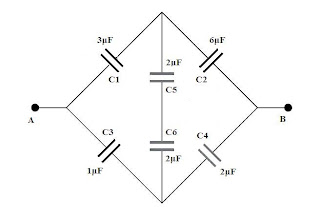## Pages

`“Life is like riding a bicycle.  To keep your balance you must keep moving.”–Albert Einstein`

## Friday, September 13, 2013

### AP Physics B & C - Electrostatics - Multiple Choice Practice Questions

“Iron rusts from disuse, stagnant water loses its purity and in cold weather becomes frozen; so does inaction sap the vigors of the mind.”
– Leonardo da Vinci

Questions on electrostatics were discussed on many occasions on this site. You can access them by clicking on the label ‘electrostatics’ below this post or by trying a search for ‘electrostatics’ using the search box provided on this page. Today we shall discuss a few more questions in this section.
(1) Right angled triangle ABC is located in a uniform electric field E. Sides AC and BC have lengths 0.5 m and 0.3 m respectively and BC is at right angles to the electric field lines (Fig.). If the electric potential difference between A and C is 80 V, what is the magnitude of the electric field E?
(a) 80 V/m
(b) 160 V/m
(c) 200 V/m
(d) 100 V/m
(e) 16 V/m
The electric potential at the point B is the same as that at the point C (since the straight line BC is at rjght angles to the direction of the uniform electric field E). Therefore the potential difference between points A and B is 80 V. The electric field E is directed along AB and hence the magnitude of E is (80 V)/(0.4 m) = 200 V/m.
[AB = √(AC2 – BC2) = √(0.52 – 0.32)  = 0.4 m]
(2) In the above question, what is the component of electric field along the direction AC?
(a) 80 V/m
(b) 160 V/m
(c) 200 V/m
(d) 100 V/m
(e) 40 V/m
The potential difference between the points A and C is 80 V and the distance between these points is 0.5 m. Therefore, the component of electric field along the direction AC is (80 V)/(0.5 m) = 160 V/m.

(3) A cube of side a has a charge Q at its centre (Fig.). What is the electric flux through one
(a) Q/ε0a
(b) Qa/ε0
(c) Q/6ε0
(d) Q/8ε0
(e)0/6
The electric flux over a closed surface, according to Gauss theorem, is Q/ε0 where Q is the net charge enclosed by the surface and ε0 is the permittivity of free space.
[You can understand the above even without knowing Gauss law. You know (from inverse square law) that the electric field at distance r from a point charge Q is Q/4πε0r2. The electric field at any point is the electric flux through unit area held with the plane of the area perpendicular to the electric field lines. Imagine a spherical surface of radius r such that the charge Q is at the centre. Since the area of the spherical surface is 4πr2 the electric field at any point on the surface must be Ф/4πr2 where Ф is the total electric flux produced by the charge Q. Therefore we have
Q/4πε0r2 = Ф/4πr2
This gives Ф = Q/ε0 as stated in Gauss law.]
Since the charge Q is at the centre of the cube, all the six faces of the cube receive equal electric flux so that the flux through one face of the cube is Ф/6 = Q/6ε0(4) The effective capacitance between terminals A and B in the network shown in the adjoining figure is
(a) 16 μF
(b) 8 μF
(c) 6 μF
(d) 16/3 μF
(e) 8/3 μF
Since the capacitors C1, C2, C3 and C4 are of values satisfying the condition C1/C2 = C3/C4, the junction of C1 and C2 is at the same potential as the junction of C3 and C4 (as in the case of a balanced Wheatstone brige). Therefore the capacitors C5 and C6 connected across the diagonal of the bridge have no effect and can be ignored.
The network therefore simplifies to the series combination of C1 and C2 connected in parallel with the series combination of C3 and C4.
Series combined value of C1 and C2 = (3×6)/(3+6) = 2 μF
Series combined value of C3 and C4 = (1×2)/(1+2) = 2/3 μF

The capacitors C1 and C4 in the above question are short circuited and the circuit then gets modified as shown in the figure. What is the effective capacitance between the terminals A and B in this situation?
(a) 11 μF
(b) 9 μF
(c) 8 μF
(d) 7.5 μF
(e) 6.5 μF
Have a careful look at the circuit. You will find that one plate of C2, C3 and C5 is connected to terminal A. The other plate of C2 as well as C3 is connected to terminal B while that of C5 is connected 6through C6 to terminal B. The series combination of C5 and C6 gives an effective capacitance of 1μF. Thus we have three capacitances 1μF, 1μF and 6μF connected in parallel across the terminals A and B.

Therefore the effective capacitance between terminals A and B in this case is 1μF + 1μF + 6μF = 8μF.